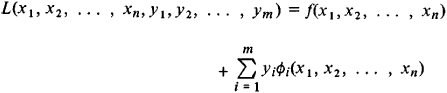Lagrange's Method of Multipliers

Lagrange’s Method of Multipliers

a method for the solution of extremum problems with side conditions. The method consists in reducing such a problem to that of finding the extreme values of a suitable auxiliary function.

If the extremum problem involves the function f(x1, x2, …, xn) and the variables are restricted by the side conditions φi (x1, x2, …, xn) = 0, for i = l, 2, …, m, then the auxiliary function isIf the auxiliary function L is differentiable and the quantities x1, x2, … xn, y1, y2 …, ym are a solution of the system of equationswhich determine the stationary values of L, then under sufficiently general assumptions, x1,x2, …,xn yield an extreme value of f. The function L is also used in the calculus of variations and in mathematical programming. Lagrange’s method of multipliers was first proposed by J. Lagrange in 1797 in connection with problems of the differential calculus.

REFERENCE

Kudriavtsev, L. D. Matematicheskii analiz, vol. 2. Moscow, 1970.
Site: Follow: Share:
Open / Close